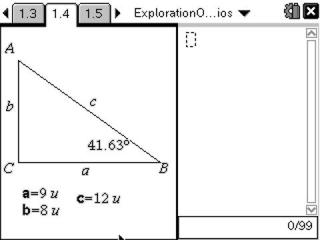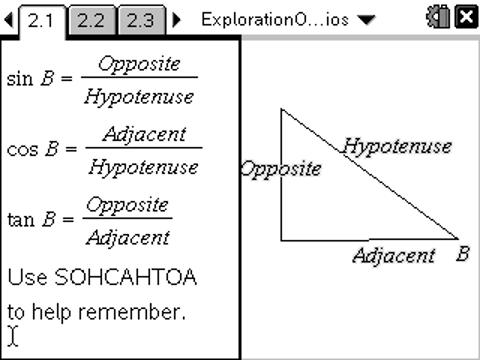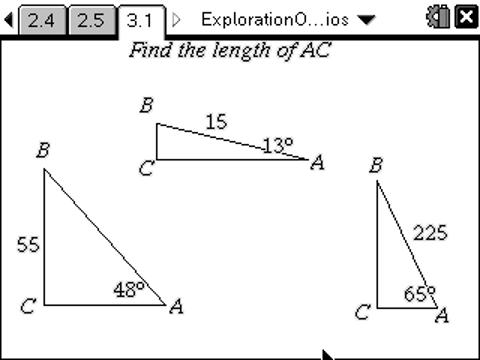# Activities

••• ##### Subject Area

• Math: Precalculus: Trigonometry (Triangle and Circular Functions)

• ##### Author9-12

20 Minutes

• ##### Device
• TI-Nspire™ Navigator™
• TI-Nspire™
• TI-Nspire™ CAS
• ##### Software

TI-Nspire™
TI-Nspire™ CAS

2.0

## Exploration of Trig Ratios#### Activity Overview

Students discover the relationship between the trigonometric functions sine, cosine, and tangent and the side length ratios of a right triangle.

#### Key Steps

•Students first investigate ratios and trigonometric values.

They find the sine, cosine, and tangent of an angle then find the ratio between the hypotenuse, adjacent and opposite sides.

•Students then explore trigonometric relationships further by completing exercises provided. These exercises ask students to find the length of a missing side of a right triangle using the trigonometric ratios.

•By the end of this activity, students will be able to use in context hypotenuse, opposite, and adjacent.

Students will know how to use trigonometric formulas to calculate a missing side length.